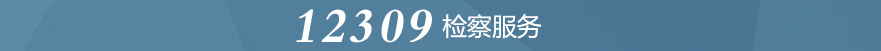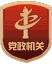6月24日，芦山县人民检察院党组书记、检察长冯松到太平镇钟灵村，与基层党员干部和人民群众面对面交流，宣讲习近平总书记来川视察重要指示精神和省第十二次党代会精神。
• []
• []
• []
• []
• []
• []
• []

“携手落实‘两法’共护祖国未来” 芦山县检察院联合多部门开展检察开放日活动

• []
• []
• []
• []
• []
• []
• []

• []
• []
• []
• []
• []
• []
• []

• []
• []
• []
• []
• []
• []
• []• []
• []
• []
• []
• []
• []
• []

• []
• []
• []
• []
• []
• []
• []